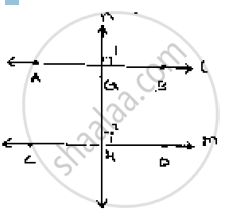# If L, M, N Are Three Lines Such that L || M and N ⊥ L, Prove that N ⊥ M. - Mathematics

If l, m, n are three lines such that l || m and n ⊥ l, prove that n ⊥ m.

#### SolutionGiven l  || ,  m ,n perpendicular l
To prove: n ⊥ m
Since l || m and n intersects them at G and H respectively
∴∠1= ∠2            [Corresponding angles]
But, U = 90°    [n ⊥ l ]
⇒ ∠2  = 90°
Hence n perpendicular m

Concept: Concept of Parallel Lines
Is there an error in this question or solution?

#### APPEARS IN

RD Sharma Mathematics for Class 9
Chapter 10 Lines and Angles
Exercise 10.4 | Q 17 | Page 48